# AP Calculus BC : Alternating Series with Error Bound

## Example Questions

### Example Question #2999 : Calculus Ii

Determine whether the following series converges or diverges: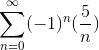The series (absolutely) converges

The series may (absolutely) converge, diverge, or conditionally converge

The series diverges

The series conditionally converges

The series (absolutely) converges

Explanation:

Given just the harmonic series, we would state that the series diverges. However, we are given the alternating harmonic series. To determine whether this series will converge or diverge, we must use the Alternating Series test.

The test states that for a given series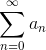where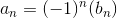or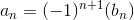where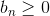for all n, if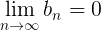and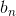is a decreasing sequence, thenis convergent.

First, we must evaluate the limit ofas n approaches infinity: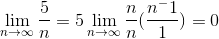The limit equals zero because the numerator of the fraction equals zero as n approaches infinity.

Next, we must determine ifis a decreasing sequence.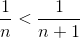, thus the sequence is decreasing.

Because both parts of the test passed, the series is (absolutely) convergent.

### Example Question #3004 : Calculus Ii

Determine whether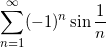converges or diverges, and explain why.

Divergent, by the test for divergence.

Divergent, by the comparison test.

Convergent, by the-series test.

More tests are needed.

Convergent, by the alternating series test.

Convergent, by the alternating series test.

Explanation:

We can use the alternating series test to show thatconverges.

We must have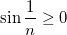for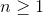in order to use this test. This is easy to see because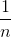is in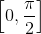for all(the values of this sequence are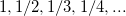), and sine is always nonzero whenever sine's argument is in.

Now we must show that

1.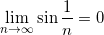2.is a decreasing sequence.

The limit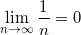implies that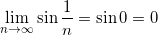so the first condition is satisfied.

We can show that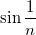is decreasing by taking its derivative and showing that it is less thanfor: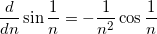The derivative is less than, because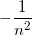is always less than, and that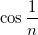is positive for, using a similar argument we used to prove thatfor. Since the derivative is less thanis a decreasing sequence. Now we have shown that the two conditions are satisfied, so we have proven thatconverges, by the alternating series test.

### Example Question #3005 : Calculus Ii

For the series: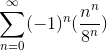, determine if the series converge or diverge.  If it diverges, choose the best reason.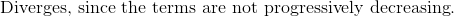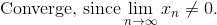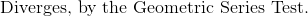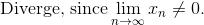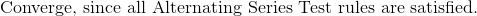Explanation:

The series given is an alternating series.

Write the three rules that are used to satisfy convergence in an alternating series test.

For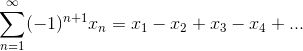: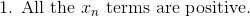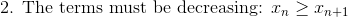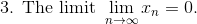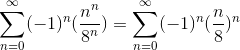The first and second conditions are satisfied since the terms are positive and are decreasing after each term.

However, the third condition is not valid since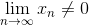and instead approaches infinity.### Example Question #3 : Alternating Series

Determine whether the series converges or diverges: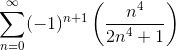The series is divergent.

The series may be convergent, divergent, or conditionally convergent.

The series is conditionally convergent.

The series is (absolutely) convergent.

The series is divergent.

Explanation:

To determine whether the series converges or diverges, we must use the Alternating Series test, which states that for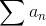- and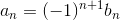wherefor all n - to converge,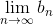must equal zero andmust be a decreasing series.

For our series,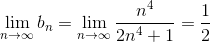because it behaves like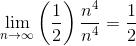.

The test fails because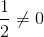so we do not need to check the second condition of the test.

The series is divergent.

### Example Question #1 : Alternating Series With Error Bound

Which of the following series does not converge?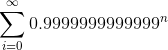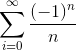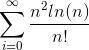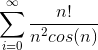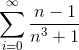Explanation:

We can show that the seriesdiverges using the ratio test.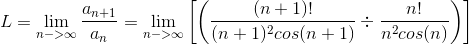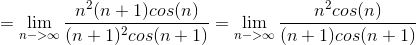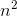will dominate over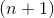since it's a higher order term. Clearly, L will not be less than, which is necessary for absolute convergence.

Alternatively, it's clear that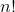is much greater than, and thus havingin the numerator will make the series diverge by the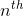limit test (since the terms clearly don't converge to zero).

The other series will converge by alternating series test, ratio test, geometric series, and comparison tests.